Area of combination of figures : circle based

Chapter 12 Class 10 Areas related to Circles
Concept wise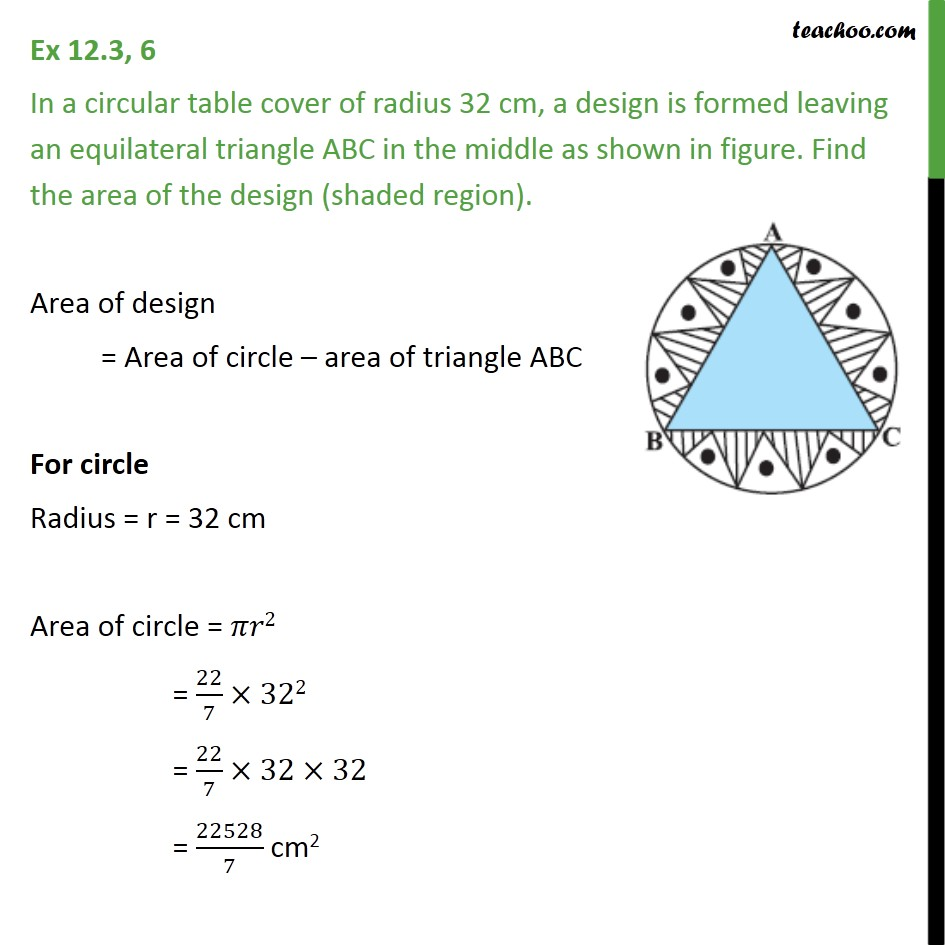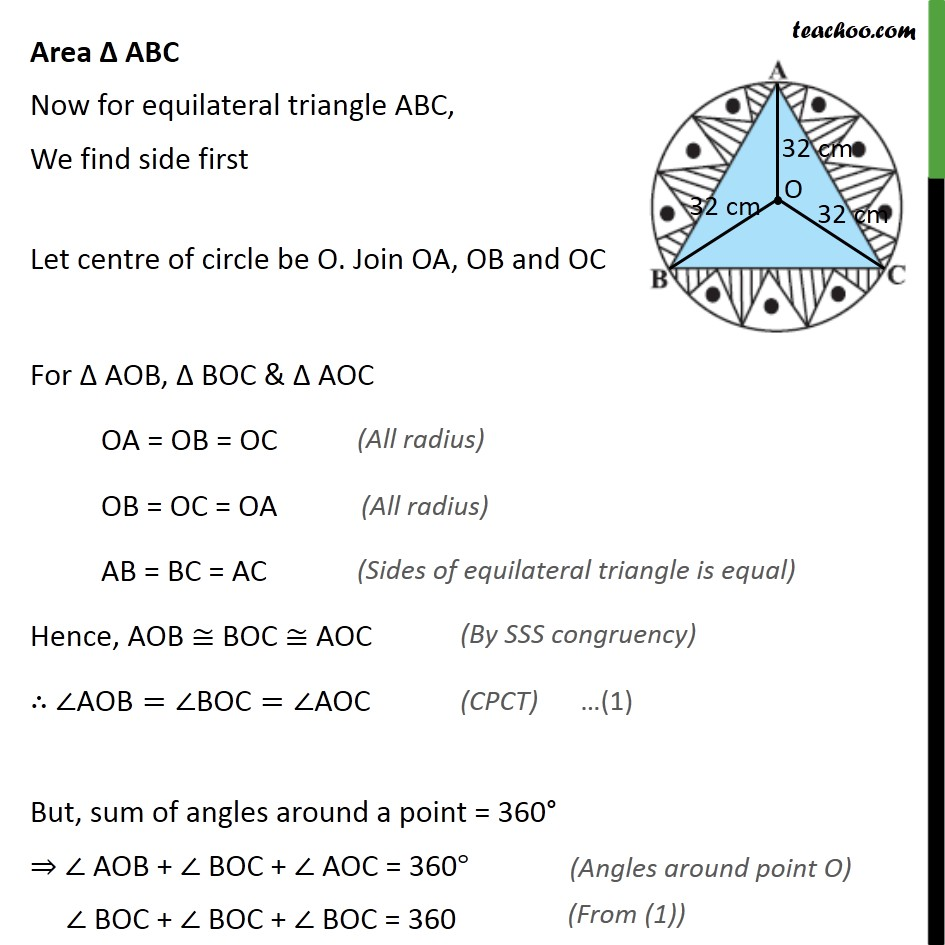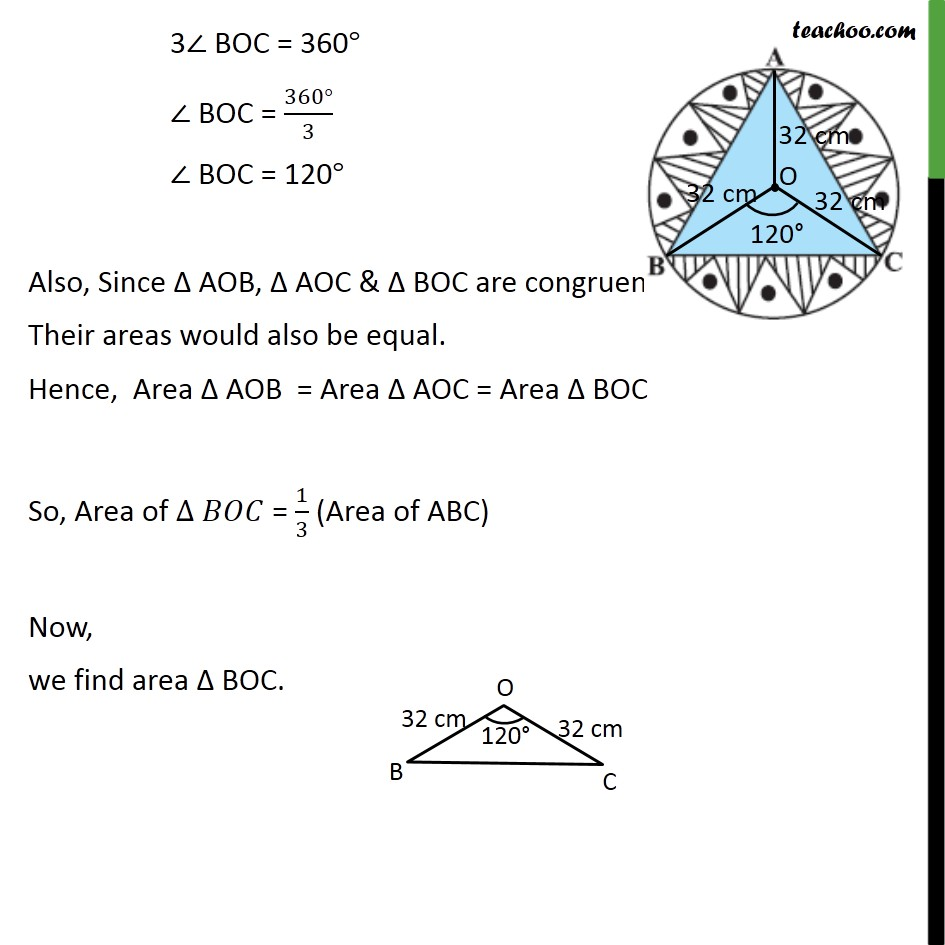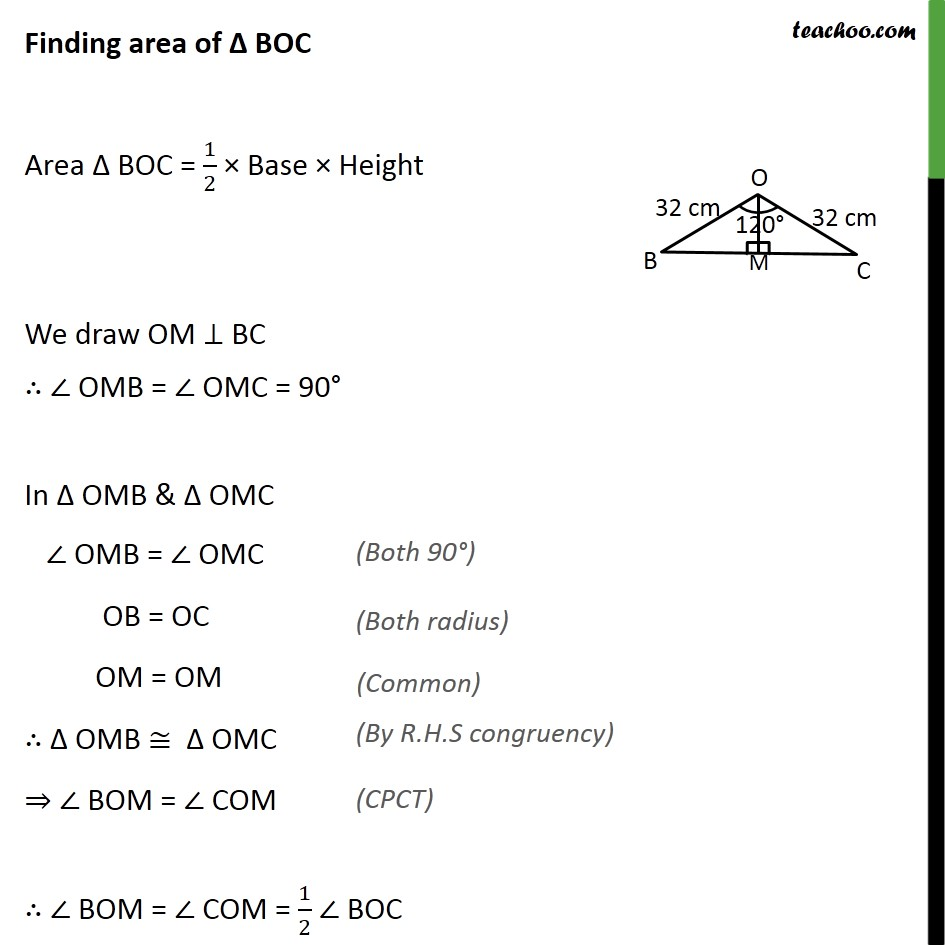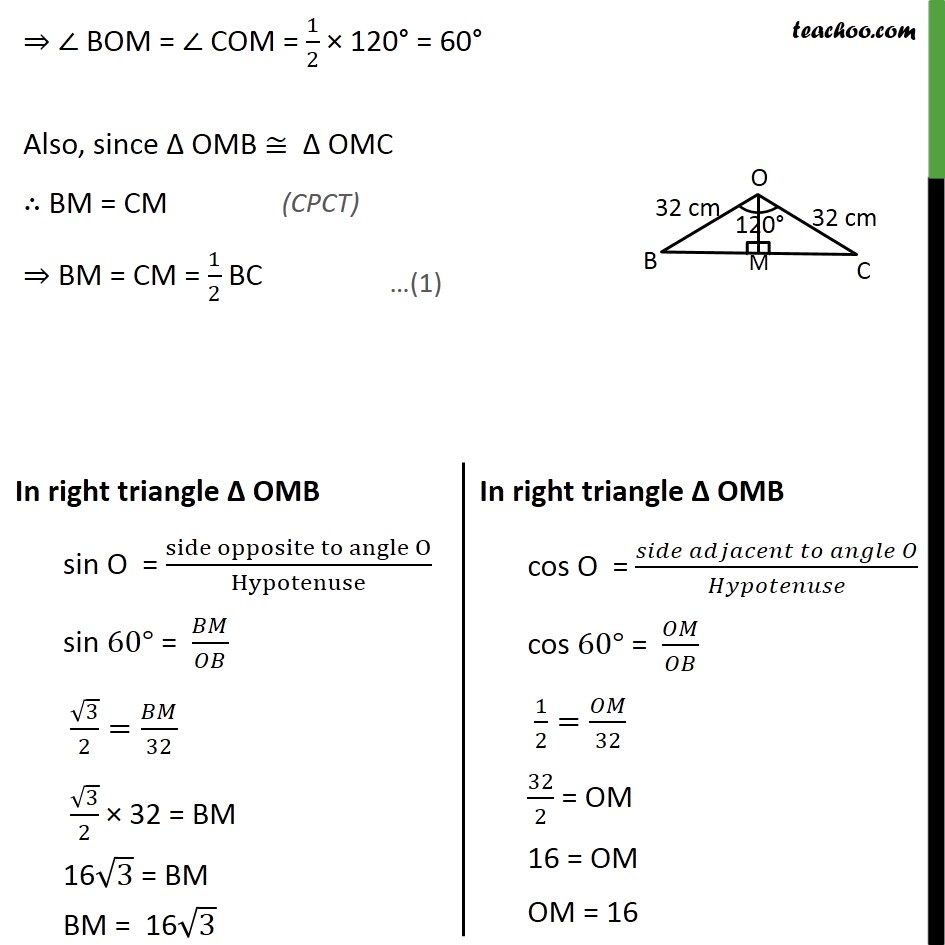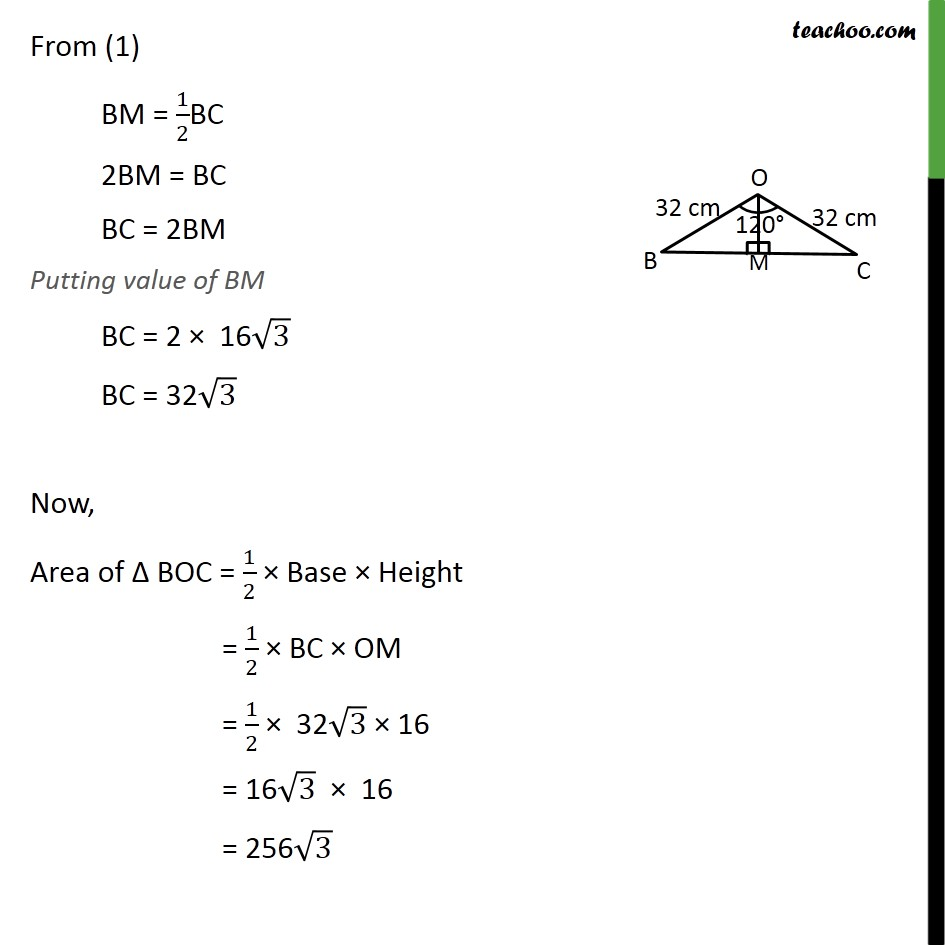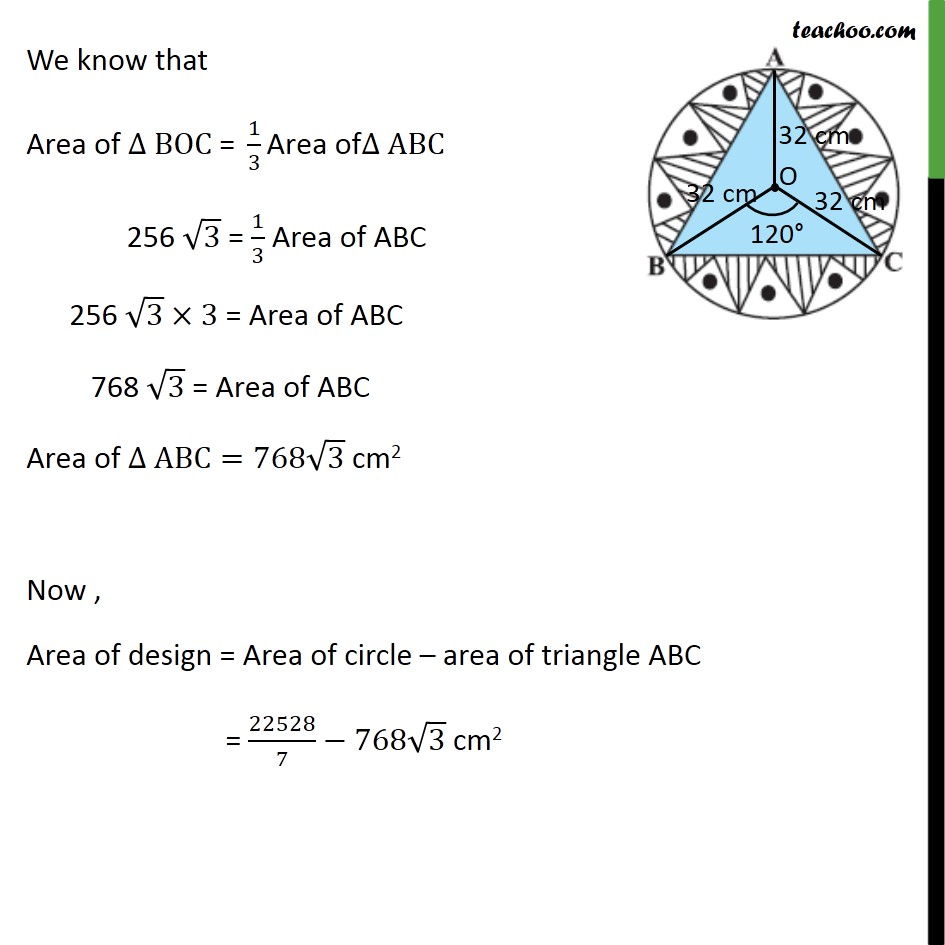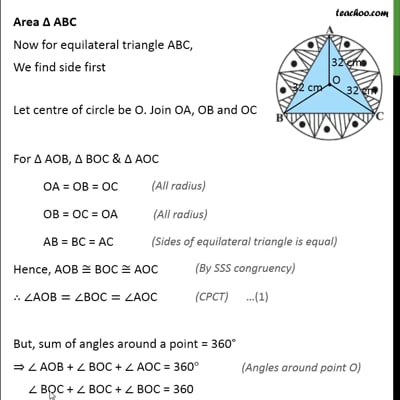This video is only available for Teachoo black users

Solve all your doubts with Teachoo Black (new monthly pack available now!)

### Transcript

Ex 12.3, 6 In a circular table cover of radius 32 cm, a design is formed leaving an equilateral triangle ABC in the middle as shown in figure. Find the area of the design (shaded region). Area of design = Area of circle – area of triangle ABC For circle Radius = r = 32 cm Area of circle = 𝜋𝑟2 = 22/7×322 = 22/7×32×32 = 22528/7 cm2 Area Δ ABC Now for equilateral triangle ABC, We find side first Let centre of circle be O. Join OA, OB and OC For Δ AOB, Δ BOC & Δ AOC OA = OB = OC OB = OC = OA AB = BC = AC Hence, AOB ≅ BOC ≅ AOC ∴ ∠"AOB"=∠"BOC"=∠AOC But, sum of angles around a point = 360° ⇒ ∠ AOB + ∠ BOC + ∠ AOC = 360° ∠ BOC + ∠ BOC + ∠ BOC = 360 3∠ BOC = 360° ∠ BOC = (360°)/3 ∠ BOC = 120° Also, Since Δ AOB, Δ AOC & Δ BOC are congruent Their areas would also be equal. Hence, Area Δ AOB = Area Δ AOC = Area Δ BOC So, Area of ∆ 𝐵𝑂𝐶 = 1/3 (Area of ABC) Now, we find area Δ BOC. Finding area of Δ BOC Area Δ BOC = 1/2 × Base × Height We draw OM ⊥ BC ∴ ∠ OMB = ∠ OMC = 90° In Δ OMB & Δ OMC ∠ OMB = ∠ OMC OB = OC OM = OM ∴ Δ OMB ≅ Δ OMC ⇒ ∠ BOM = ∠ COM ∴ ∠ BOM = ∠ COM = 1/2 ∠ BOC ⇒ ∠ BOM = ∠ COM = 1/2 × 120° = 60° Also, since Δ OMB ≅ Δ OMC ∴ BM = CM ⇒ BM = CM = 1/2 BC From (1) BM = 1/2BC 2BM = BC BC = 2BM Putting value of BM BC = 2 × 16√3 BC = 32√3 Now, Area of Δ BOC = 1/2 × Base × Height = 1/2 × BC × OM = 1/2 × 32√3 × 16 = 16√3 × 16 = 256√3 We know that Area of ∆ BOC" = " 1/3 "Area of" ∆ ABC 256 √3 = 1/3 Area of ABC 256 √3×3 = Area of ABC 768 √3 = Area of ABC Area of ∆ ABC=768√3 cm2 Now , Area of design = Area of circle – area of triangle ABC = 22528/7−768√3 cm2